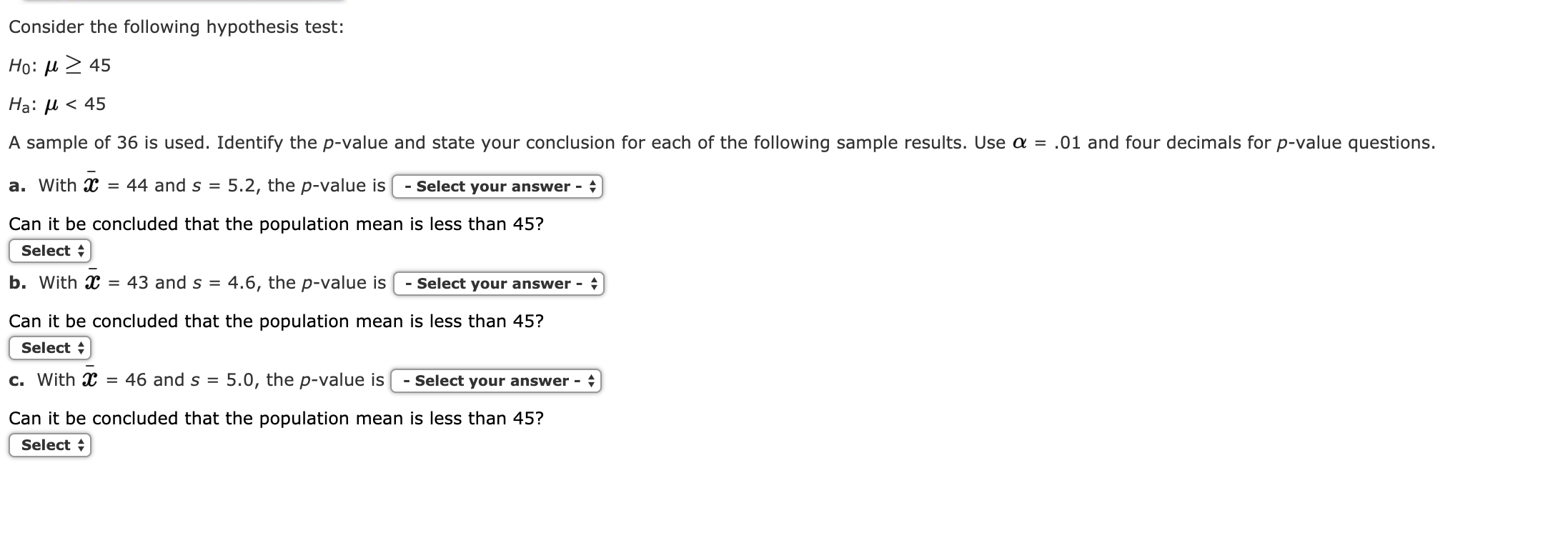# Consider the following hypothesis test: Ho: μ> 45 Ha: μ < 45 A sample of 36 is used. Identify the p-value and state your conclusion for each of the following sample results. Use α-.01 and four decimals for p-value questions a. with X = 44 and s = 5.2, the p-value is-select your answer- Can it be concluded that the population mean is less than 45? Select b. with X = 43 and S-4.6, the p-value is | -select your answer Can it be concluded that the population mean is less than 45? Select c. with X = 46 and s-5.0, the p-value is l -select your answer Can it be concluded that the population mean is less than 45? Select

Questionhelp_outlineImage TranscriptioncloseConsider the following hypothesis test: Ho: μ> 45 Ha: μ < 45 A sample of 36 is used. Identify the p-value and state your conclusion for each of the following sample results. Use α-.01 and four decimals for p-value questions a. with X = 44 and s = 5.2, the p-value is-select your answer- Can it be concluded that the population mean is less than 45? Select b. with X = 43 and S-4.6, the p-value is | -select your answer Can it be concluded that the population mean is less than 45? Select c. with X = 46 and s-5.0, the p-value is l -select your answer Can it be concluded that the population mean is less than 45? Select fullscreen

### Want to see this answer and more?

Experts are waiting 24/7 to provide step-by-step solutions in as fast as 30 minutes!*

*Response times vary by subject and question complexity. Median response time is 34 minutes and may be longer for new subjects.
Tagged in
MathStatistics

### Hypothesis Testing